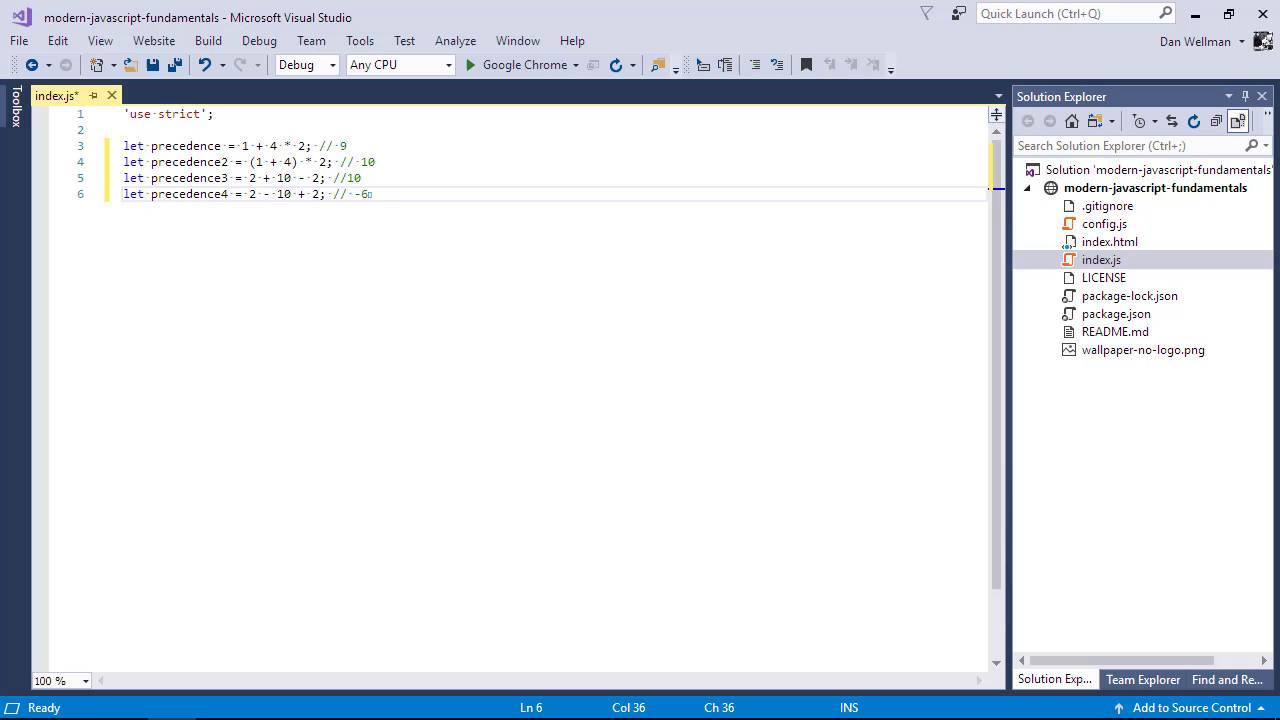FREELessons: 65Length: 7.1 hours• Overview
• Transcript

# 2.5 Operator Precedence

Not all operators are created equal! In this lesson we'll see the order in which operators are evaluated when more than one type of operator is used in an expression.

## 11.Conclusion1 lesson, 00:43

### 2.5 Operator Precedence

Hi, Folks. In this lesson, we're going to look at one more aspect of operators, and that is something called operator precedence. Which just means the order in which operators are evaluated when there are multiple operators in the same expression. So let's say that we want to perform the following calculation We might think that we're adding 1 to 4, which gives 5, and then multiplying it by 2 to give 10, right? Wrong, the multiplication operator has a higher precedence than the addition operator, and so this is evaluated first. The calculation that we actually perform is 4 multiplied by 2 + 1, which gives 9. If we wanted to perform the calculation we thought that we were performing, we could wrap the first part in parentheses. Parenthesis, which are also known as the grouping operator, have the highest precedence of any other operator. So any part of a calculation enclosed within parentheses will automatically be evaluated first. If we use two operators of equal precedence, these are usually evaluated left to right. Sometimes however, operators of equal precedence are evaluated from right to left. The order in which operators of the same precedence are evaluated is known as operator associativity. Out of the operators that we've looked at so far following parentheses, the highest weighted operators are the post fix, increment and decrement operators, followed by not and the unary operators, these are all evaluated from right to left. Following this are the arithmetic operators, which are evaluated in the order multiplication, division, modulus, addition, and lastly subtraction. Next in terms of precedence are the comparison operators, these are all evaluated from left to right. And following this are the logical operators and all the assignment operator, the spread operator, and last of all the comma operator, which has the lowest precedence out of all the available operators. These are all evaluated right to left except for the comma operator, which is left to right associative. These rules are fairly complex and sometimes hard to remember, especially when you're just beginning with JavaScript. For now, I wouldn't worry about them too much. But at some point when you're more comfortable with JavaScript, I'd recommend that you look at the developer documentation on precedence and associativity up on the Mozilla Developer Network. So in this lesson, we learned all about operator precedence and associativity in JavaScripts focusing on the operators that we've covered in the course. In the next lesson, we're going to take a quick look at reserved words and identify names. Thanks for watching.

Back to the top